Function Repository Resource:

# GeoCirclePoints

Get geo coordinates equally spaced around a circle centered at a GeoLocation

Contributed by: Jon McLoone
 ResourceFunction["GeoCirclePoints"][loc,dist,n] gives the positions of n points equally spaced at a distance dist around the GeoLocation loc. ResourceFunction["GeoCirclePoints"][loc,{dist1,dist2},n] gives the positions of n points at equal angles around the ellipse with semiaxes of lengths dist1 and dist2 around the GeoLocation loc. ResourceFunction["GeoCirclePoints"][loc,{dist1,dist2,align},n] gives the positions of n points on the ellipse whose major axis is at the angle align.

## Examples

### Basic Examples (1)

Find 10 points at a 10 mile radius from Oxford:

 In:=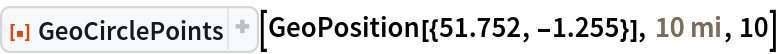Out=In:=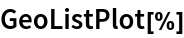Out=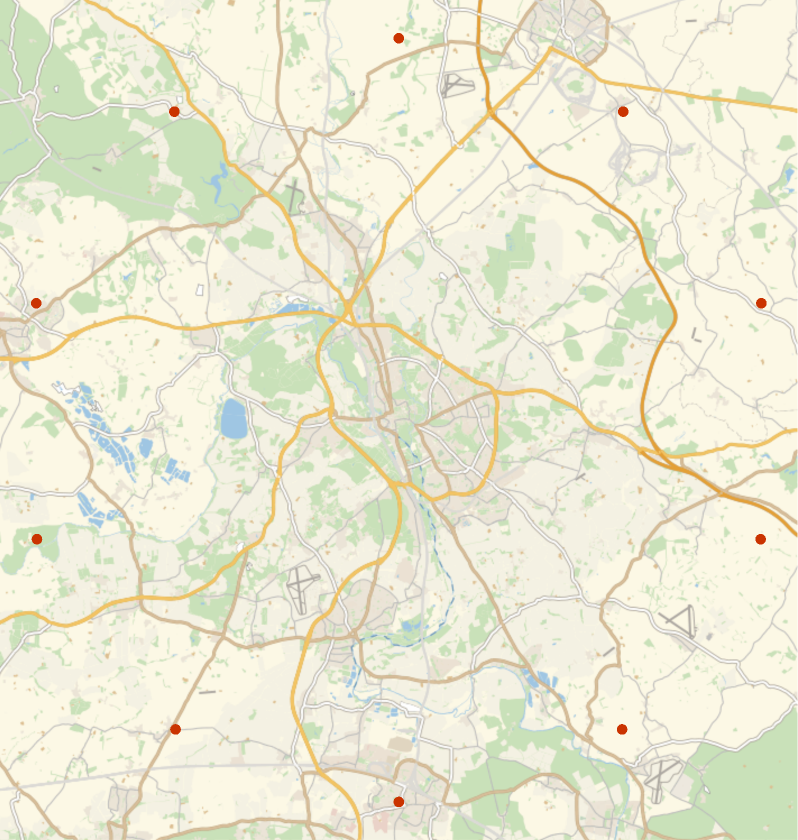### Scope (3)

GeoCirclePoints can accept an Entity with a geo position property:

 In:=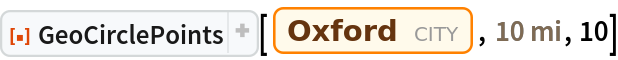Out=Provide two semiaxes lengths to generate points on an ellipse:

 In:=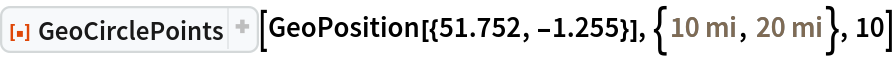Out=In:=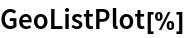Out=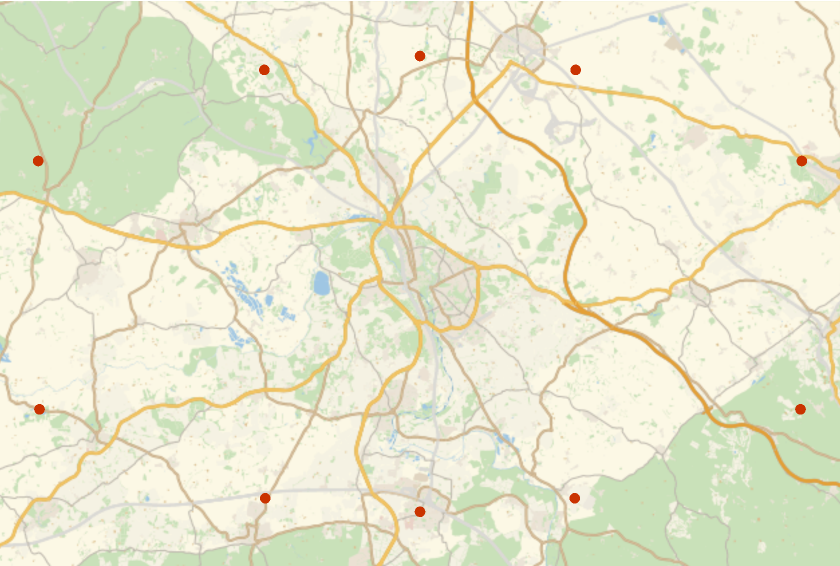Ellipses are aligned to the equator unless you provide an alignment angle:

 In:=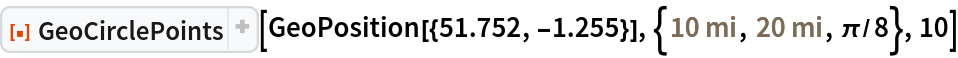Out=In:=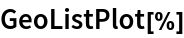Out=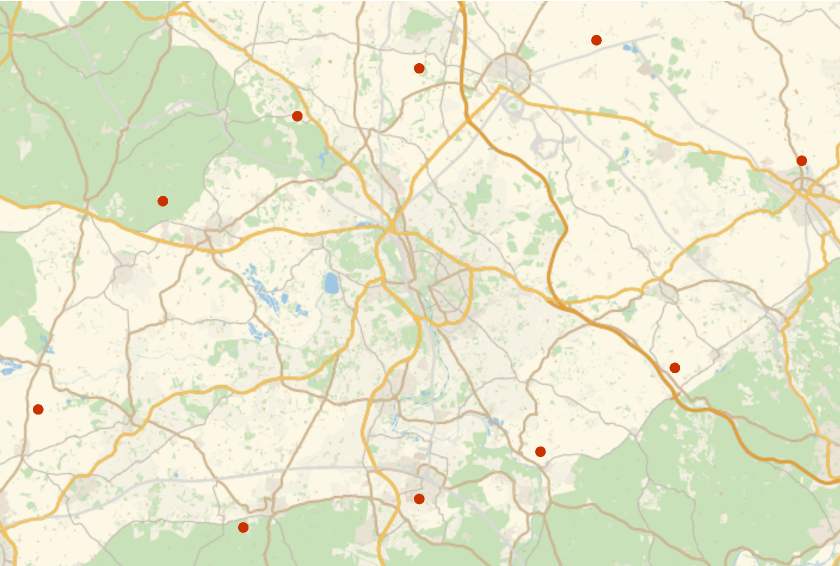### Properties and Relations (2)

All points have equal geodesic distance from the circle center:

 In:=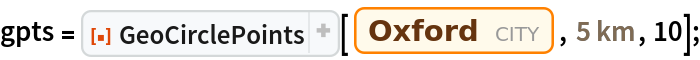In:=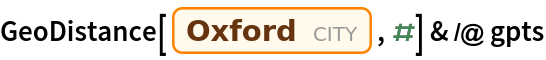Out=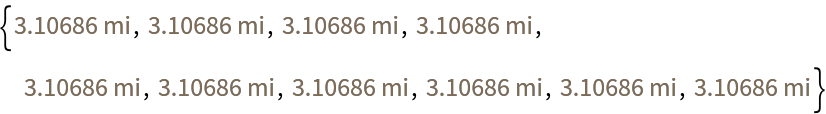All points are at equal distances from their neighbors:

 In:=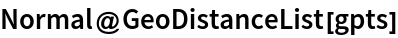Out=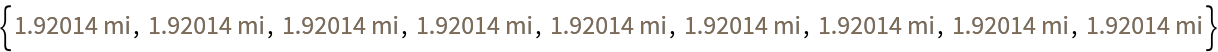Jon McLoone

## Version History

• 1.1.0 – 08 August 2022
• 1.0.0 – 11 January 2022